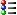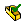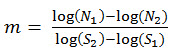Applying a MaterialRemoving a MaterialCreating a Custom MaterialAdding Sustainability Data to a Custom MaterialEditing a Custom MaterialManaging Favorite MaterialsCreating a Material LibraryMaterial Dialog Box - Properties TabMaterial Dialog Box - Appearance TabMaterial Dialog Box - CrossHatch TabMaterial Dialog Box - Custom TabMaterial Dialog Box - Application Data TabMaterial Dialog Box - Favorites TabMaterial Dialog Box - Fatigue SN Curves TabMaterial Dialog Box - Tables & Curves TabAccess to SOLIDWORKS Materials Web PortalGlossary

# Material Dialog Box - Fatigue SN Curves Tab

Use the Fatigue SN Curves tab to define SN curves for a fatigue study. SN curves are used in fatigue studies only.

## Source

To display this dialog box:

Open a part, right-click Materialin the FeatureManager design tree, and select Edit Material.

The Fatigue SN Curves tab is only available when SOLIDWORKS Simulation is added in.

A few materials in the SOLIDWORKS material library have fatigue SN curves already defined. These materials are identified by a suffix (SN) attached to the end of their names in the material list of the material library. The reference of the SN curves is the Atlas of Fatigue Curves, ASM International.

Sets the interpolation scheme and the source of the data.
 Interpolate Sets the scheme for interpolating alternating stresses against the number of cycles of SN curve. Log-log. Logarithmic interpolation (base 10) for the number of cycles and the alternating stress. This option is recommended for SN curves with few data points that are widely scattered on both axes unless the curve is better fit by another interpolation scheme. Semi-log. Linear interpolation for stress and logarithmic for number of cycles. This option is recommended for SN curves with relatively small stress range compared to variation in the number of cycles unless the curve is better fit by another interpolation scheme. Linear. Linear interpolation for both stress and number of cycles. This option is recommended for SN curves with many data points unless the curve is better fit by another interpolation schemeExample of SN Interpolation Schemes In some cases, the SN curve is available in the form of an equation that is obtained by fitting the experimental data. In such cases, if the equation matches one of the interpolation schemes, it may be adequate to define only the extremes of the curve as data points and use the proper interpolation scheme. Define Define curve data manually. Select from up to ten curves in the menu. Derive from material elastic modulus Derive the SN curve automatically based on ASME SN curves and the moduli of elasticity of the reference material and the active material. The source SN shows in the Preview area. Based on ASME Austenitic Steel curves Based on ASME Carbon Steel curves The SN curve is derived by dividing each stress value of the reference SN curve by the modulus of elasticity of the reference ASME material and multiplying it by the modulus of elasticity of the current material. The associated numbers of cycles remain unchanged. Define fatigue S-N equation (for random vibration fatigue analysis) Available for fatigue studies that are based on results from dynamic random vibration studies.

## Table data

Lists curve data. Available only when Define is selected in the Source box.
 Stress Ratio (R) Available only when Custom defined is selected under Select material source. Units Alternating stress units.If you are typing values in, make sure to select the desired units that you want to use before entering the values. Curve data table Enter pairs of number of cycles and alternating stress values if you are defining the curve manually. If the curve is derived based on a reference ASME SN curve, the scaled curve is listed.You can use copy and paste to populate the table. File Imports curve data from a file. Available only when Custom defined is selected under Select material source. View Displays a graph of the current data in the table. Delete Deletes the selected data row. Available only when Custom defined is selected under Select material source.
To apply the same SN curve to bodies with different materials, in the fatigue study tree right-click Parts, and select Apply Fatigue Data to All Bodies.

## S-N Curve Equation (Basquin Equation)

Available for fatigue studies based on results from linear dynamic - random vibration studies.

 Estimate Basquin constants from S-N curve Select to let the program calculate the Basquin equation constants based on the given data points of the S-N curve. For high cycle fatigue (number of cycles N > 104) an S-N curve can be approximated by a straight line when plotted on a log-log scale. Basquin’s equation is a power law relationship between the applied stress cycles (S) in the y-axis and the number of cycles to failure (N) in the x-axis. Basquin’s equation is only valid up to the endurance limit or fatigue limit. The endurance limit is the highest alternating stress that does not result in fatigue failure. In other words, if the alternating stress is equal to or lower than the endurance limit, the number of stress cycles to cause failure becomes very large (practically infinite). The endurance limit is usually defined for zero-mean alternating stresses. The endurance limit is also called the fatigue limit. Some metals do not have a measurable endurance limit. The program considers the stress range values Sr for the Basquin equation. It multiplies by 2 the alternating stress values (Sa) of the S-N curve to account for a fully-reversed load (Sr = 2*Sa). The Basquin’s equation of the S-N fatigue curve is given as: N = B / (Sr) m , or log (Sr) = B - m log (N) When you select this option the Curve specific constant (B) and Slope of S-N curve (m) options are inactive. The software calculates the B and m constants by fitting a straight line through the S-N data points using the least-squares line fitting technique. These constants are material parameters determined by fatigue testing experiments under different stress levels. Units Sets the units for the stress range Sr. Consider the cut-off point for the S-N curve at row Sets the cut-off point of the S-N curve that is considered for the calculation of the Basquin constants. If you enter 0 or 1, all the data points of the S-N curve are considered for fitting a straight line using the least-square fitting method. For example, if you enter 4, the data set of the first four rows of the S-N curve are considered for fitting a straight line and calculating the Basquin constants B and m. It is recommended to use as a cut-off point the fatigue endurance limit. The S-N curve is considered a horizontal line after the cut-off point. Curve specific constant (B) Enter the value ( must be positive real number) for the S-N specific constant B. This is the value of the stress range Sr at one cycle. Slope of S-N curve (m) Enter the value (must be a positive integer) for the slope of the S-N curve m. When two data points (N1, S1) and (N2, S2) are known, the slope of the S-N curve m is given by:Source Enter information about the source of the S-N data. View Select to view a graph of the S-N curve. Save Saves the S-N curve data in a *.dat or *.csv (comma delimited) file format.

Provide feedback on this topic

* Required

 *Email: Subject: Feedback on Help Topics Page: Material Dialog Box - Fatigue SN Curves Tab *Comment: * I acknowledge I have read and I hereby accept the privacy policy under which my Personal Data will be used by Dassault Systèmes

Print Topic

Select the scope of content to print:

x

We have detected you are using a browser version older than Internet Explorer 7. For optimized display, we suggest upgrading your browser to Internet Explorer 7 or newer.

Never show this message again
x

Web Help Content Version: SOLIDWORKS 2015 SP05

To disable Web help from within SOLIDWORKS and use local help instead, click Help > Use SOLIDWORKS Web Help.

To report problems encountered with the Web help interface and search, contact your local support representative. To provide feedback on individual help topics, use the “Feedback on this topic” link on the individual topic page.